Definition Of Similar Figures

Similar figures are figures that have the same shape but not necessarily the same size.

Any two corresponding sides in two similar figures have a common ratio called the scale factor.The trapezoids ABCD and EFGH shown above are similar. So,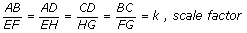The ratio of areas of two similar figures is the square of the scale factor.
The ratio of volumes of two similar figures is the cube of the scale factor.

Example of Similar Figures

Find the value of x, if the figures are similar.

Solved Example on Similar Figures

Ques: Find the number of Similar Figures in the measurement 4.07 cm.

Choices: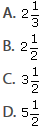Step 2:[Write the proportion.]
Step 3: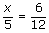[Substitute the values.]
Step 5:[Divide both sides by 12.]
Step 6: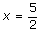[Simplify.]
Step 7: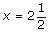[Convert improper fraction into mixed number.]
Step 8: The value of x is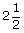.